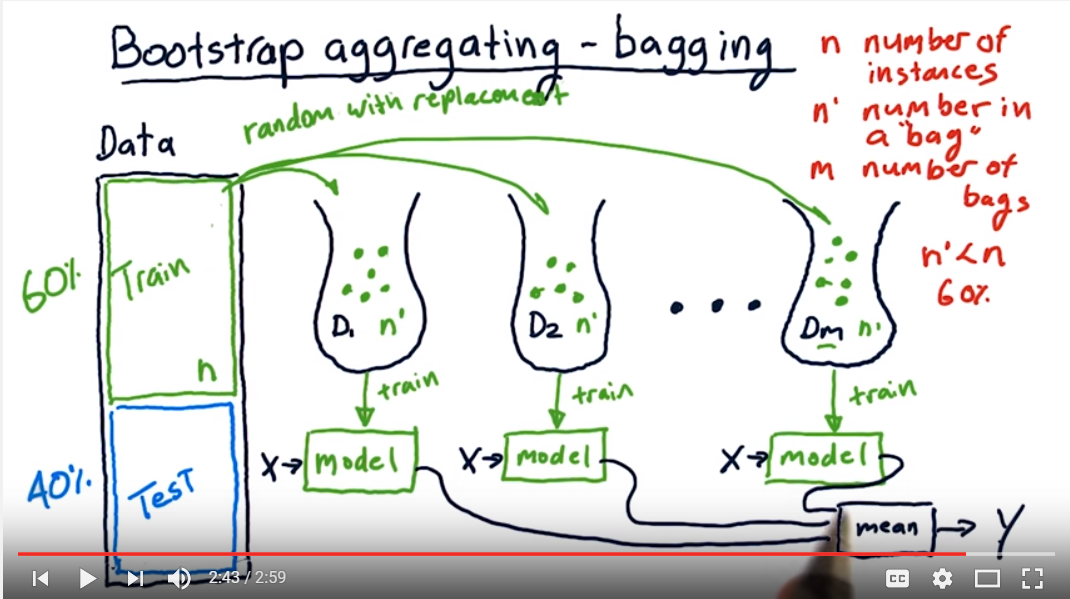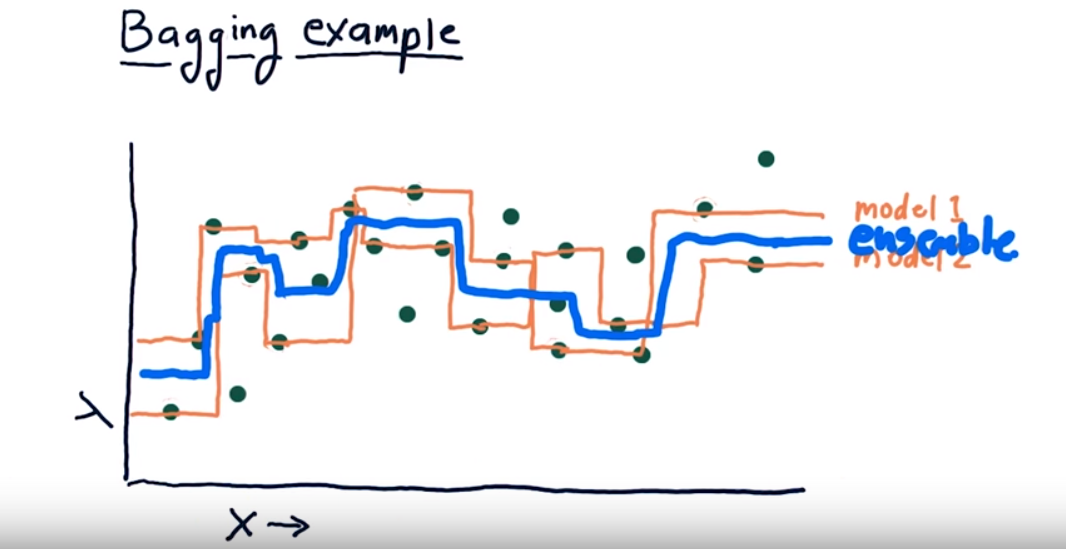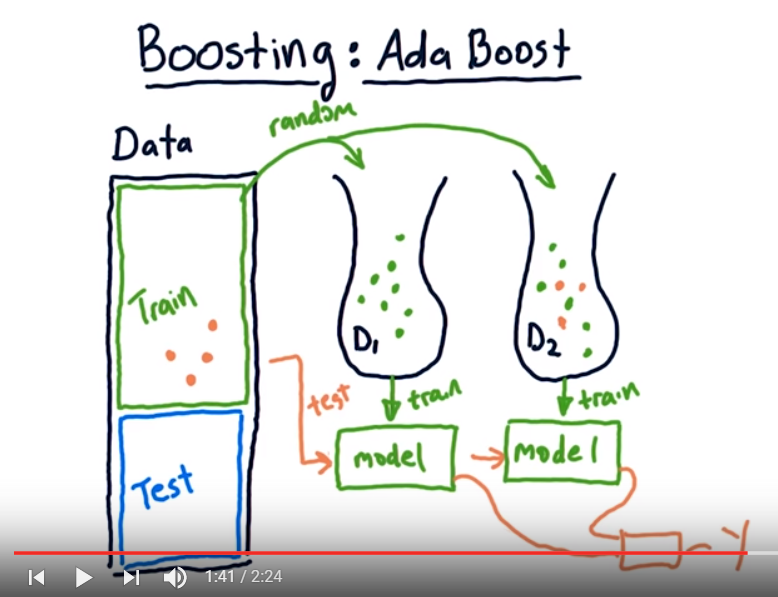Comment on page

# Ensembles

1. 4.
Machine learning Mastery on
1. 1.
1. 1.
Stacked Generalization Ensemble
2. 2.
Multi-Class Classification Problem
3. 3.
Multilayer Perceptron Model
4. 4.
Train and Save Sub-Models
5. 5.
Separate Stacking Model
6. 6.
Integrated Stacking Model
2. 2.
1. 1.
Plurality Voting.
2. 2.
Majority Voting.
3. 3.
Unanimous Voting.
4. 4.
Weighted Voting.
3. 3.
1. 1.
Voting Ensembles
2. 2.
Weighted Average
3. 3.
Blending Ensemble
4. 4.
Super Learner Ensemble
4. 4.
Dynamic Ensemble Selection (DES) for Classification in Python - Dynamic Ensemble Selection algorithms operate much like DCS algorithms, except predictions are made using votes from multiple classifier models instead of a single best model. In effect, each region of the input feature space is owned by a subset of models that perform best in that region.
1. 1.
k-Nearest Neighbor Oracle (KNORA) With Scikit-Learn
1. 1.
KNORA-Eliminate (KNORA-E)
2. 2.
KNORA-Union (KNORA-U)
2. 2.
Hyperparameter Tuning for KNORA
1. 1.
Explore k in k-Nearest Neighbor
2. 2.
Explore Algorithms for Classifier Pool
5. 5.
1. 1.
Mixture of Experts
1. 1.
2. 2.
Expert Models
3. 3.
Gating Model
4. 4.
Pooling Method
2. 2.
Relationship With Other Techniques
1. 1.
Mixture of Experts and Decision Trees
2. 2.
Mixture of Experts and Stacking
6. 6.
Strong Learners vs. Weak Learners in Ensemble Learning - Weak learners are models that perform slightly better than random guessing. Strong learners are models that have arbitrarily good accuracy. Weak and strong learners are tools from computational learning theory and provide the basis for the development of the boosting class of ensemble methods.
2. 7.
1. 1.
Basic Ensemble Techniques
2. 2.
2.1 Max Voting
3. 3.
2.2 Averaging
4. 4.
2.3 Weighted Average
5. 5.
6. 6.
3.1 Stacking
7. 7.
3.2 Blending
8. 8.
3.3 Bagging
9. 9.
3.4 Boosting
10. 10.
Algorithms based on Bagging and Boosting
11. 11.
4.1 Bagging meta-estimator
12. 12.
4.2 Random Forest
13. 13.
14. 14.
4.4 GBM
15. 15.
4.5 XGB
16. 16.
4.6 Light GBM
17. 17.
4.7 CatBoost

### ​Ensembles in WEKA

- bagging (random sample selection, multi classifier training), random forest (random feature selection for each tree, multi tree training), boosting(creating stumps, each new stump tries to fix the previous error, at last combining results using new data, each model is assigned a skill weight and accounted for in the end), voting(majority vote, any set of algorithms within weka, results combined via mean or some other way), stacking(same as voting but combining predictions using a meta model is used).

### BAGGING - bootstrap aggregating

Bagging - best example so far, create m bags, put n’<n samples (60% of n) in each bag - with replacement which means that the same sample can be selected twice or more, query from the test (x) each of the m models, calculate mean, this is the classification.Overfitting - not an issue with bagging, as the mean of the models actually averages or smoothes the “curves”. Even if all of them are overfitted.### BOOSTING

Mastery on using all the boosting algorithms: Gradient Boosting with Scikit-Learn, XGBoost, LightGBM, and CatBoost
Adaboost: similar to bagging, create a system that chooses from samples that were modelled poorly before.
1. 1.
create bag_1 with n’ features <n with replacement, create the model_1, test on ALL train.
2. 2.
Create bag_2 with n’ features with replacement, but add a bias for selecting from the samples that were wrongly classified by the model_1. Create a model_2. Average results from model_1 and model_2. I.e., who was classified correctly or not.
3. 3.
Create bag_3 with n’ features with replacement, but add a bias for selecting from the samples that were wrongly classified by the model_1+2. Create a model_3. Average results from model_1, 2 & 3 I.e., who was classified correctly or not. Iterate onward.
4. 4.
Create bag_m with n’ features with replacement, but add a bias for selecting from the samples that were wrongly classified by the previous steps.### XGBOOST

R Installation in Weka, then XGBOOST in weka through R
Parameters for weka mlr class.xgboost.
• Here is an example configuration for multi-class classification:
• weka.classifiers.mlr.MLRClassifier -learner “nrounds = 10, max_depth = 2, eta = 0.5, nthread = 2”
• classif.xgboost -params "nrounds = 1000, max_depth = 4, eta = 0.05, nthread = 5, objective = \"multi:softprob\"
Copy: nrounds = 10, max_depth = 2, eta = 0.5, nthread = 2
#Random Forest™ - 1000 trees bst <- xgboost(data = train\$data, label = train\$label, max_depth = 4, num_parallel_tree = 1000, subsample = 0.5, colsample_bytree =0.5, nrounds = 1, objective = "binary:logistic") #Boosting - 3 rounds bst <- xgboost(data = train\$data, label = train\$label, max_depth = 4, nrounds = 3, objective = "binary:logistic")
RF1000: - max_depth = 4, num_parallel_tree = 1000, subsample = 0.5, colsample_bytree =0.5, nrounds = 1, nthread = 2
XG: nrounds = 10, max_depth = 4, eta = 0.5, nthread = 2